# Place Value Worksheet for Grade 3

When starting with mathematics kids are taught that place value refers to the value of each digit in any given number.  For example: In the number 1239, 9 represents ones or unit value, 3 represents 3 tens 0r 30, 2 represents 2 hundred or 200 and 1 represents 1 thousand or 1000. As simple as this definition seems, the importance of understanding this is magnanimous.

Unless a student understands the concept of place values, he can’t differentiate between the value and magnitude of numbers properly since it is only when he learns that a number with a digit in tens place value is bigger than a single-digit number and so on, he would be able to tell that 100 is greater than 50.

At this point, there seems no further need to elaborate on why learning place values is important. Without the proper knowledge of this concept, students won’t be able to perform any arithmetic calculations such as multiplication, division, subtraction, or addition for that matter.

## Place Value Practice WorksheetsThe following worksheet brings several place-value-related exercises for students to practice and get familiar with it.  The worksheet is meant for third graders and by the time a student is in the third grade, he is usually familiar with the concept. There are several different types of questions that test his understanding and problem-solving capabilities.

In the first worksheet, there are eight different questions. In the first two questions, students have to identify the largest and smallest number from the given numbers. Then there are some after and before exercises in which they have to write the numbers that come before or after the given number. Finally, there are questions asking them to find the sum and difference of two numbers.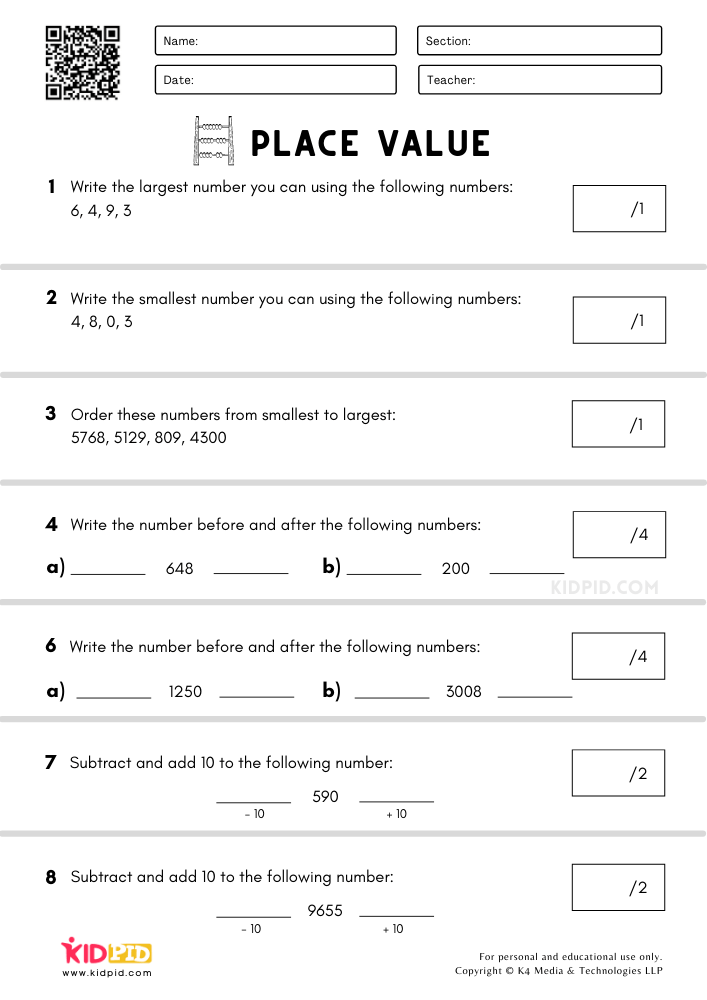As mentioned earlier, it is only by understanding place values that one can tell the magnitude of a number. The next sheet asks students to identify which of the two numbers is greater. They have to draw the greater than or less than symbol between each number set. For example, 520 is smaller than 600, hence they’ll mark the “<” sign (smaller than sign) between the number set (520 < 600).  Similarly in the second exercise, all they need to write if the number on the left side is greater than or smaller than the one on the right side.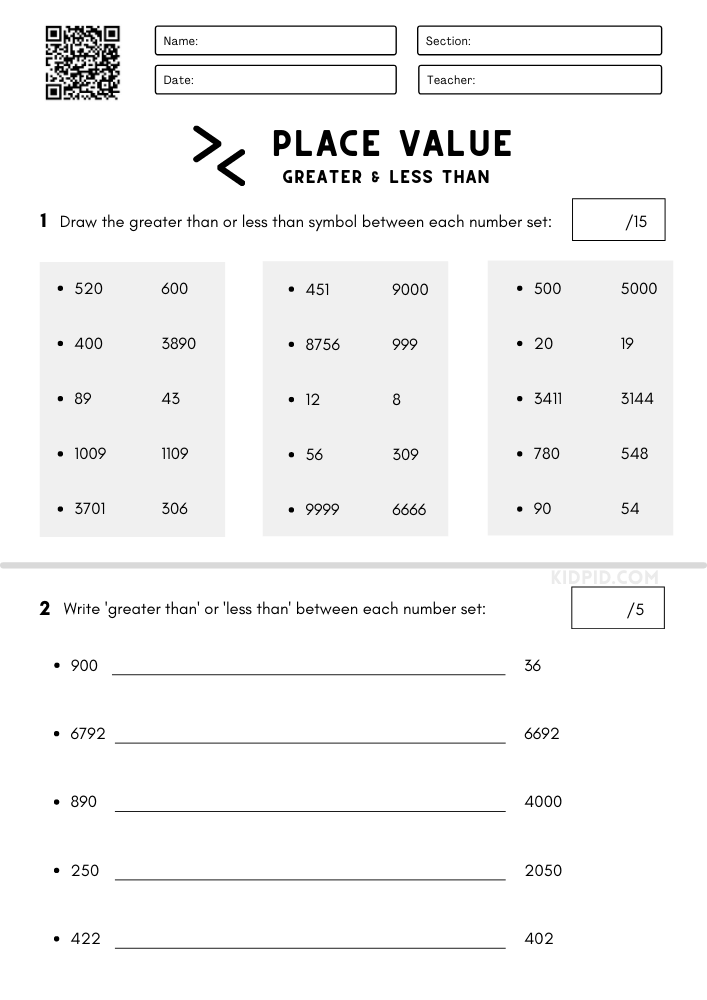In the following sheet, students have to round the given numbers to the nearest ten (10), hundred (100), and thousand (1000).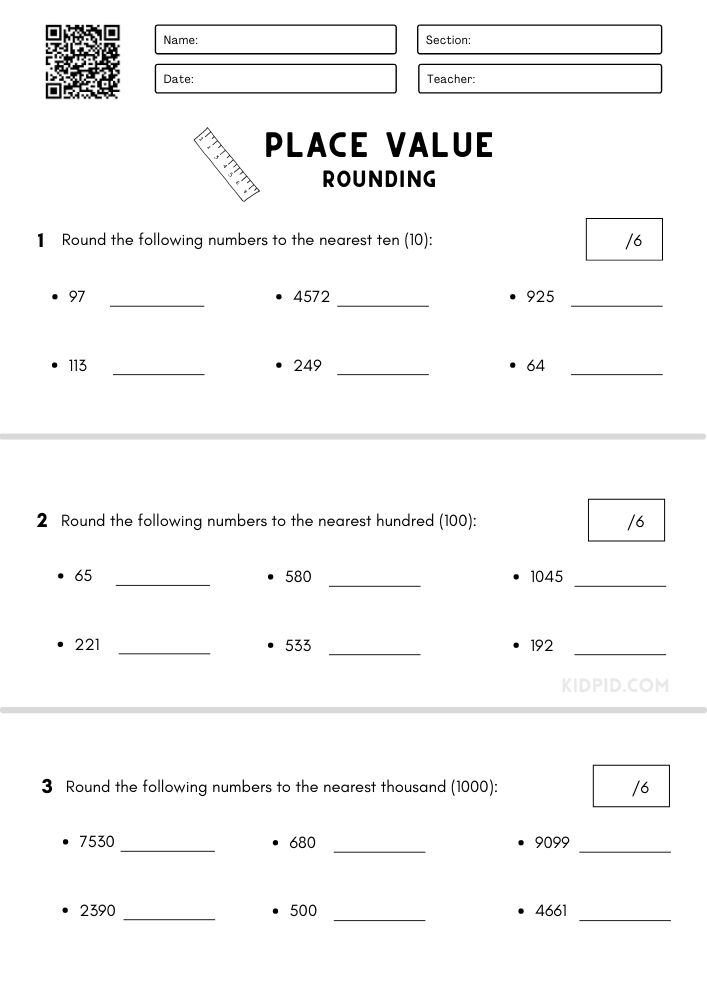The fourth sheet provides three relatively simpler exercises. In the first question, students need to state the place value of the underlined digit in the numbers. In the next question, they have to partition the given numbers and in question number three, they are required to write the numbers in numerals.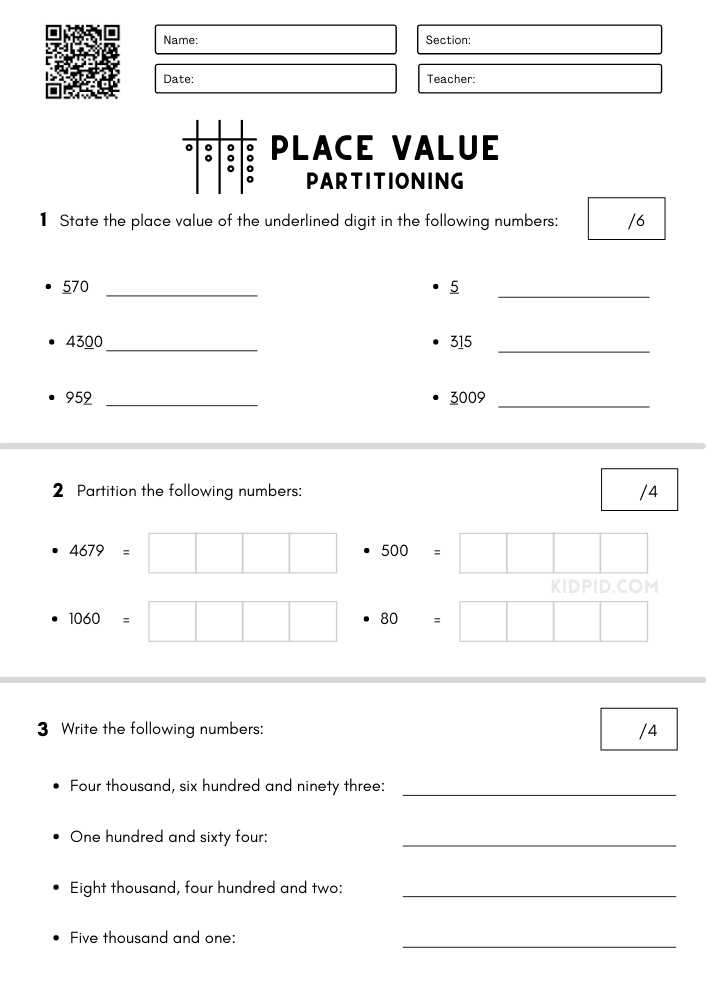The final sheet consists of questions that require students to count forward and backward by tens and one hundred.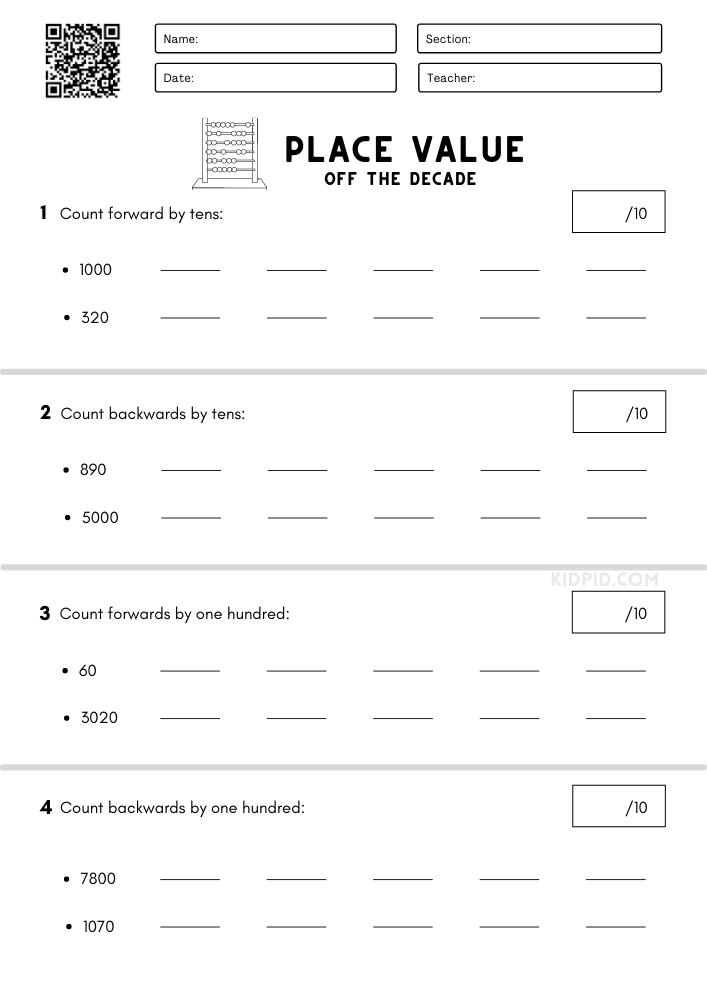The worksheet covers various topics all of which can be solved only with the knowledge of place values. It’ll ensure that students excel in basic mathematical functions and develop computational skills with time as they move on to more complex arithmetic operations. Download the worksheet, print it, and get your students learning!

## Single-digit Addition Math Worksheets & Free Printables

We have bought single-digit addition math foundational worksheets that will help your kid in building and brushing their counting skills. In this busy world, it…

## Count Backward and Write the Number Worksheets for Kindergarten

Enhance your kid’s knowledge with some count backward worksheet as these will help your kid to master arranging the numbers in descending order. We have…

## Colorful Rainbow Crafts for Kids

Craftwork is not limited to kids, in fact, it’s not confined to any age limit. By doing craftwork our creativity increases. We are in school,…

## Easy Apple Craft Ideas For Kids

We all know about apples, it’s a fruit which we all have eaten once or more than a thousand times. It has so many benefits…

## Times Tables Multiplication Matching Card Game Flashcards

Multiplication is one of the four foundational operations in mathematics. Students are taught multiplication from a very young age since it is essential for them…

+